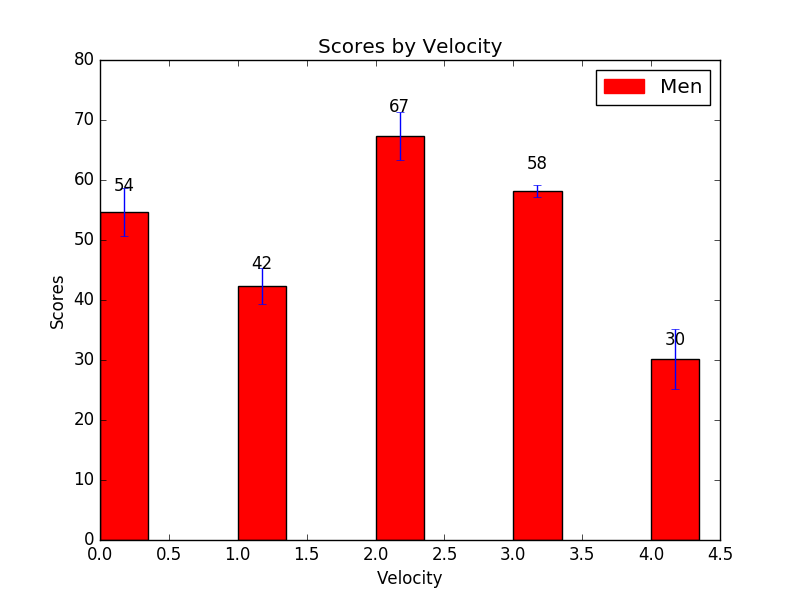﻿ Matplotlib Bar Chart: Create bar plots with errorbars on the same figure and attach a text label above each bar - w3resource# Matplotlib Bar Chart: Create bar plots with errorbars on the same figure and attach a text label above each bar

## Matplotlib Bar Chart: Exercise-13 with Solution

Write a Python program to create bar plots with errorbars on the same figure. Attach a text label above each bar displaying men means (integer value).

Sample Date
Mean velocity: 0.2474, 0.1235, 0.1737, 0.1824
Standard deviation of velocity: 0.3314, 0.2278, 0.2836, 0.2645

Sample Solution:

Python Code:

``````import numpy as np
import matplotlib.pyplot as plt
import matplotlib.patches as mpatches

N = 5
men_means = (54.74, 42.35, 67.37, 58.24, 30.25)
men_std= (4, 3, 4, 1, 5)

ind = np.arange(N)  # the x locations for the groups
width = 0.35       # the width of the bars

fig, ax = plt.subplots()
rects1 = ax.bar(ind, men_means, width, color='r', yerr=men_std)

# add some text for labels, title and axes ticks
plt.ylabel('Scores')
plt.xlabel('Velocity')
plt.title('Scores by Velocity')

red_patch = mpatches.Patch(color='red', label='Men')
plt.legend(handles=[red_patch])

def autolabel(rects):
"""
Attach a text label above each bar displaying its height
"""
for rect in rects:
height = rect.get_height()
ax.text(rect.get_x() + rect.get_width()/2., 1.05*height,
'%d' % int(height),
ha='center', va='bottom')
autolabel(rects1)
plt.show()
```
```

Sample Output:Python Code Editor: